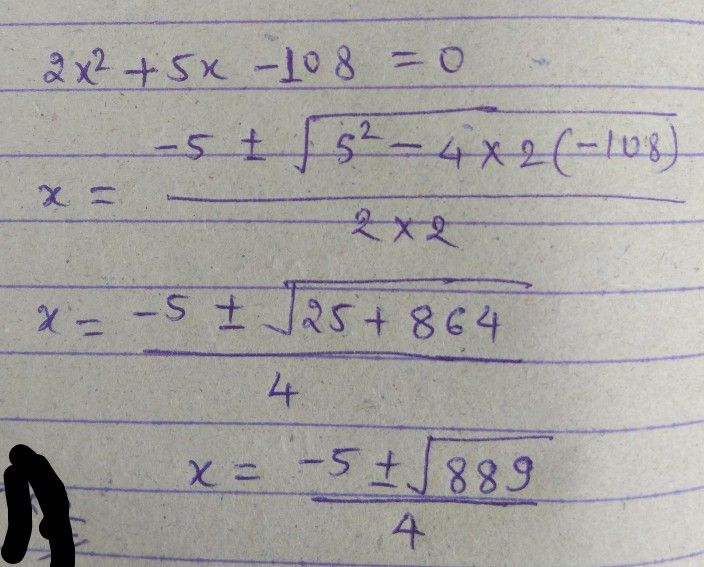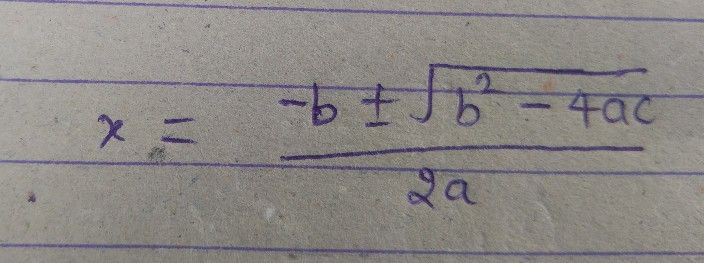Symbol
Problem$x^{2}+5x-10\sqrt{}$ $=$ $-\tarc{C}$
7th-9th grade
Algebra
Question content
2x^2 +5x-108=0
SolutionQanda teacher - SuneelStudent
is that in quadratic equation?Qanda teacher - Suneel
Yes, from Shri Dharacharya Formula.Student
ok thank youQanda teacher - SuneelStudent
Thanks